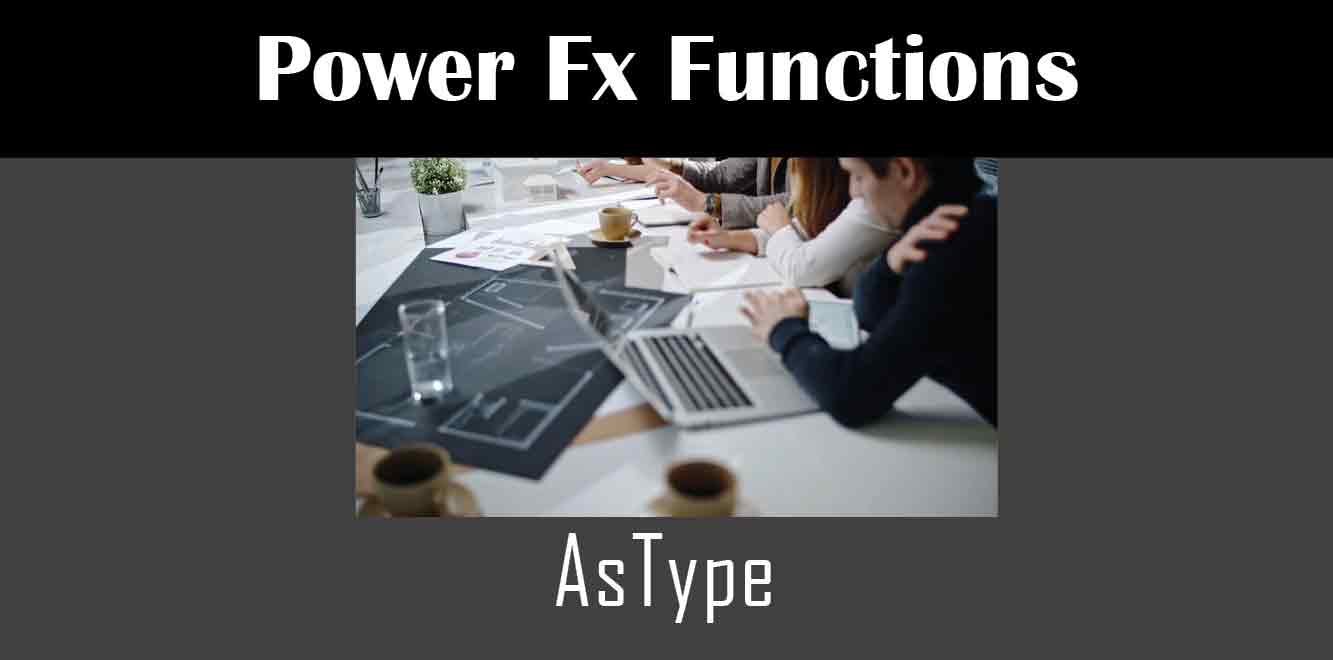# AsType

## YOne of the most useful functions in Power Fx is the AsType function. This function allows you to convert values from one data type to another, which can be incredibly handy when working with different data sources and APIs.

In this article, we’ll take a closer look at how to use the AsType function in Power Apps, including some real-world examples of how it can be used in your applications.

## What is the AsType Function?

The AsType function in Power Fx is used to convert a value from one data type to another. For example, you might use the AsType function to convert a string value to a number value, or vice versa.

The syntax for the AsType function is as follows:

``` AsType(value, target_type) ```

– Value: The value that you want to convert.

– Target_type: The target data type that you want to convert the value to.

Here’s a simple example of how to use the AsType function:

``` AsType("42", Number) ```

This would convert the string “42” to a number value of 42.

## Using AsType in Power Apps

Now that we understand the basics of the AsType function, let’s take a look at some practical examples of how it can be used in Power Apps.

### Example 1: Converting a Text Input to a Number

Suppose you have a text input control in your app that allows users to enter a number. However, you need to perform some calculations on that value, which requires it to be in number format.

To convert the text input to a number, you can use the AsType function as follows:

``` AsType(TextInput1.Text, Number) ```

This would convert the value of the TextInput1 control to a number value.

### Example 2: Converting a Date String to a Date Value

When working with APIs and data sources, you may encounter dates in string format. However, to perform certain operations on those dates, you’ll need to convert them to a date value.

Here’s an example of how to use the AsType function to convert a date string to a date value:

``` AsType("2022-10-31T00:00:00Z", DateTimeZone) ```

This would convert the date string “2022-10-31T00:00:00Z” to a date value.

### Example 3: Converting a Boolean Value to a Text Value

Sometimes you may need to convert a boolean value to a text value. For example, you may want to display “Yes” or “No” instead of “True” or “False”.

Here’s an example of how to use the AsType function to convert a boolean value to a text value:

``` AsType(true, Text) ```

This would convert the boolean value true to the text value “True”.

The AsType function is a powerful tool in the Power Fx formula language that allows you to convert values from one data type to another. By using this function in your Power Apps applications, you can work with different data sources and APIs more easily and efficiently.

We hope this article has been helpful in explaining how to use the AsType function in Power Apps. If you have any questions or feedback, please feel free to let us know in the comments below.